### Home > CALC > Chapter 9 > Lesson 9.1.1 > Problem9-9

9-9.
1. Examine the integrals below. Consider the multiple tools available for integrating and use the best strategy. After evaluating each integral, write a short description of your method. Homework Help ✎

1.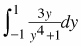2.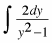3.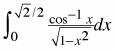4.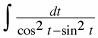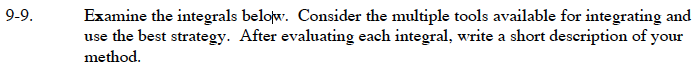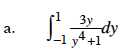Graph the function. What do you notice?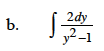Use partial fraction decomposition to rewrite the integrand as a sum of fractions.

$\frac{2}{y^2-1}=\frac{A}{y-1}+\frac{B}{y+1}$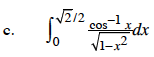Use substitution.

Let u = cos–1(x). Then du = _____.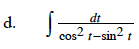Recall that cos2(x) – sin2(x) = cos(2x).

$\int\frac{1}{\cos(2t)}dt=\int\sec(2t)dt$

$=\frac{1}{2}\int\sec(u)du\text{ }u=2x$

$=\frac{1}{2}\ln(\tan(u)+sec(u))$